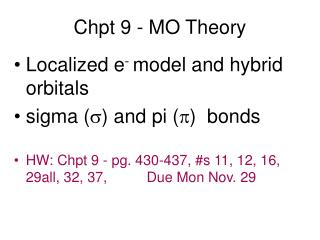DownloadDownload PresentationChpt 9 - MO Theory

# Chpt 9 - MO Theory

Download Presentation## Chpt 9 - MO Theory

- - - - - - - - - - - - - - - - - - - - - - - - - - - E N D - - - - - - - - - - - - - - - - - - - - - - - - - - -
##### Presentation Transcript

1. Chpt 9 - MO Theory • Localized e- model and hybrid orbitals • sigma () and pi () bonds • HW: Chpt 9 - pg. 430-437, #s 11, 12, 16, 29all, 32, 37, Due Mon Nov. 29

2. Covalent Bonding Recall • A bond is a combination of forces that hold groups of atoms together and make them function as a unit. • A bond will form if the energy of the aggregate is lower than that of the separated atoms.

3. Rationale for Orbital Hybridization What is shape and bond angles of CH4? What is the valence electron configuration of a carbon atom? s2p2 We now want to think of the bonding orbitals for methane as being formed by an overlap of atomic orbitals. Assume that the carbon atom has four equivalent atomic orbitals, arranged tetrahedrally.

4. Hybridization Mixing of the native atomic orbitals to form special orbitals for bonding. sp3 Hybridization • Combination of one s and three p orbitals. • Whenever a set of equivalent tetrahedral atomic orbitals is required by an atom, the localized electron model assumes that the atom adopts a set of sp3 orbitals; the atom becomes sp3 hybridized.

5. Energy diagram for sp3 An s and 3 p orbitals become 4 equal sp3 orbitals visualization

6. C2H4 example Draw the Lewis structure for C2H4 (ethylene)? • What is the shape of an ethylene molecule? trigonal planar around each carbon atom • What are the approximate bondangles around the carbon atoms? 120o

7. sp2 hybridization & Energy level diagram • Combination of one s and two p orbitals. • Gives a trigonal planar arrangement of atomic orbitals. • One p orbital is not used. • Oriented perpendicular to the plane of the sp2 orbitals can be used to form pi () bonds.

8. Ethylene C2H4 hybrid bonding Carbon atom Carbon atom

9. Ethylene sigma and pi bonding Above - sigma bonds in ethylene Above - sigma bond & pi bond on carbon in ethylene Left - total bonding in ethlene

10. sp hybridization example Draw the Lewis structure for CO2.  • What is the shape of a carbon dioxide molecule? linear • What are the bond angles? 180o

11. sp Hybridization • Combination of one s and one p orbital. • Gives a linear arrangement of atomic orbitals. • Two p orbitals are not used. • Needed to form the  bonds.

12. Sigma & Pi bonds Sigma () bond • an Electron pair is shared in an area centered on a line running between the atoms. Pi () bond • Forms double and triple bonds by sharing electron pair(s) in the space above and below the σ bond. • Uses the unhybridized p orbitals.

13. Hybrid orbitals in CO2 Yellow are 2 sp hybrid orbitals --> make  bonds to O , Blue are unhybridized p orbitals that make  bonds to O (double bonds)

14. dsp3 hybridization Draw the Lewis structure for PCl5. • What is the shape of a phosphorus pentachloride molecule? trigonal bipyramidal • What are the bond angles? 90o and 120o • Combination of one d, one s, and three p orbitals. • Gives a trigonal bipyramidal arrangement of five equivalent hybrid orbitals.

15. d2sp3 Hybridization Draw the Lewis structure for XeF4. • What is the shape of a xenon tetrafluoride molecule? octahedral • What are the bond angles? 90o and 180o • Combination of two d, one s, and three p orbitals. • Gives an octahedral arrangement of six equivalent hybrid orbitals.

16. XeF4 example

17. Examples Draw the Lewis structure for HCN. Which hybrid orbitals are used? Draw HCN: • Showing all bonds between atoms. • Labeling each bond as  or .

18. More examples hybridization Determine the bond angle and expected hybridization of the central atom for each of the following molecules: NH3 SO2 KrF2 CO2 ICl5 NH3 – 109.5o, sp3 SO2 – 120o, sp2 KrF2 – 90o, 120o, dsp3 CO2 – 180o, sp ICl5 – 90o, 180o, d2sp3

19. NO3-1 delocalized pi orbital